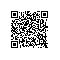# 快速排序C实现（阿里巴巴 2012年全国校招笔试题）

《快速排序C实现》 这篇文章最早是我原创，2012年发表在当时我的百度空间的一篇文章，没想到机缘巧合，此题竟然无意中被阿里巴巴选录，被改成填空题，成为当年阿里巴巴全国校招的笔试题，机缘巧合，可叹可叹！现在博客搬家，我重新把这篇文章保持原貌、原封不动从百度空间搬到CSDN新的博客。

#include <stdio.h>

/*输出*/
void show(int [],int);

void quickSort(int array[],int left,int right)
{

if(left>right)

return;

/*取最左边的值为pivot(基准)*/
int i=left,j=right,pivot=array[left];

while(i<j)
{
while( (i<j) && (pivot <= array[j]) )
j--;
if(i<j)
array[i++]=array[j];

while( (i<j) && (array[i] <= pivot) )
i++;
if(i<j)
array[j--]=array[i];
}

array[j]=pivot;/*也可以是 array[i]=piovt。因为此时i=j*/

quickSort(array,left,i-1);
quickSort(array,i+1,right);
}

void main()
{
/*测试数据*/
//int array[]={4,3,9,0,8,5,7,1,6,2};
int array[]={9,8,7,6,5,4,3,2,1,0,123,22,34,22,56,76,345,221};

int LEN=sizeof(array)/sizeof(int);

printf("原始数组:\n");
show(array,LEN);
printf("\n-----\n");

quickSort(array,0,LEN-1);

printf("\n排序结果:\n");
show(array,LEN);
}

/*打印*/
void show(int a[],int len)
{
int i;
for(i=0;i<len;i++)
printf("%d ",a[i]);
}


﻿﻿使用钉钉扫一扫加入圈子
+ 订阅